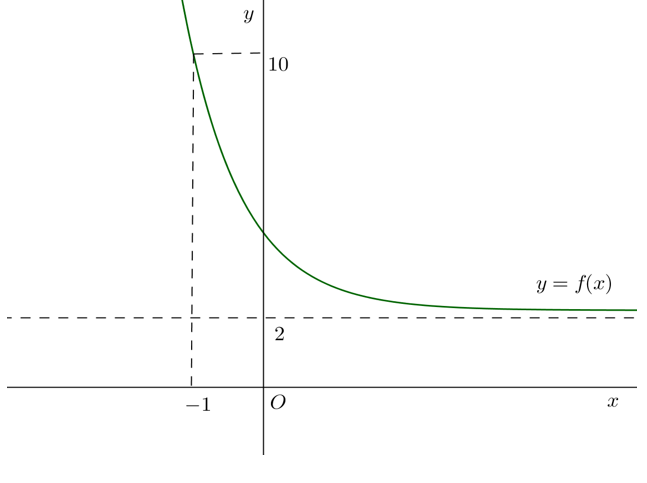Algebra

# Graphs of Exponential Functions

Which of the following could be the graph of $y = - \frac{1}{a^x},$ if $a>1 ?$Let $m$ and $n$ be the maximum and minimum values, respectively, of $y=5 ^{1-x},$ where $-1 \leq x \leq 1$. What is the value of $m-n$?

The graph of $y = 5^{-x + 3} + 7^{-x - 1} + 6$ never goes below the line $y = k.$

What is the largest possible value of $k$ such that the statement above is true?

Let $m$ and $n$ be the maximum and minimum values, respectively, of $y=\left(\frac{1}{7}\right)^{-x^2+4x-5}$ in the interval $2 \leq x \leq 3$. What is the value of $m+2n$?Shown above is the graph of $y = f(x) ,$ which is the reflection of $y = 2^{2x+a} + b$ in the $y$-axis. If the graph of $y = f(x)$ passes through $(-1, 10)$ and the equation of the graph's asymptote is $y=2$, what is $a+b$?

×